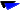# VACETS Regular Technical Column

"Science for Everyone"

"Science for Everyone" was a technical column posted regularly on the VACETS forum. The author of the following articles is Dr. Vo Ta Duc. For more publications produced by other VACETS  members, please visit the VACETS Member Publications page or Technical Columns page.

The VACETS Technical Column is contributed by various members , especially those of the VACETS Technical Affairs Committe. Articles are posted regulary on vacets@peak.org forum. Please send questions, comments and suggestions to vacets-ta@vacets.orgTue, 25 Oct 1994 Primes and FLT This is a few more suggestions for the people who are planning to find a counterexample of the Fermat's last theorem. There are many kinds of prime numbers like Fermat numbers, Mersenne numbers, Lucas numbers, etc... Those primes can be very huge. But those methods do not calculate all the possible primes and each number calculated may have to be tested and the testing process may take hours of computer time. For the purpose of searching for a solution to the FLT (or set some lower limit), we may only need to find ALL the primes smaller than n, say like all primes less than 1 trillion. Below is a method, invented by who know (I think this method was invented some thousands years ago, when people discovered prime numbers), and it guarantees to find all the primes. And it is not too slow either. Here is the FORTRAN program I just wrote to test the method. It took 160 seconds of my slugish VAX station to find all 78498 primes p < 1 million. To find all the primes below 1 trillion, I estimate it would take less than 160s * sqrt(1 trillion / 1 million) = 160000s = 44 hours on my very slow machine and I don't think that is too bad. If you want to try it then you need to modify the program to do it step by step and put the results from each step into a file. e.g., search from range 1-1million, 1million-2million, ... because I don't think any machine can have enough memory to contain all 1 trillion numbers in its memory. c-------------- integer n(500000),p(100000) nn=1000000 imax=(nn-1)/2 do i=1,imax n(i)=i*2+1 enddo p(1)=2 k=1 10 k=k+1 p(k)=n(1) ip=n(1) j=0 do 20 i=2,imax if(jmod(n(i),ip).eq.0)goto 20 j=j+1 n(j)=n(i) 20 continue imax=j if(n(1)**2.gt.n(imax))goto 40 if(imax.gt.1)goto 10 40 do 50 i=1,imax p(k+i)=n(i) 50 continue do 30 i=1,k+imax,10 write(6,900)(p(j),j=i,i+9) 30 continue write(6,*)' Total primes = ',k+imax stop 900 format(x,10i7) end -------------------- Now after the prime number problem is solved, you can start on the FLT. The problem of calculating a^n+b^n=c^n can be a problem when n is large. However, we don't have to calculate a^n, b^n, and c^n directly. You may still need a way to handle very large numbers a, b, and c, but not a^n, b^n, and c^n. The way to do it is: We now know for large n and assume b>=c, then b < c <= b(1+.693/n). Or c=b(1+x) where x <= 0.693/n = very small number for large n. This type of form gives us some advantages. 1/ We don't have to start from b=2 or 3... We can start from b>=n/.693 2/ Don't need to vary c from c=2 up. We can have c in the range b < c <= b+0.693b/n 3/ From a^n+b^n=c^n then we have a^n=c^n-b^n=b^n[(1+x)^n-1] Using Taylor expansion (You may use other kinds of expansions if you like) we then have a^n=b^n [xn + x^2 n(n-1)/2 + x^3 n(n-1)(n-2)/6 + ...] or a = b [xn + x^2 n(n-1)/2 + x^3 n(n-1)(n-2)/6 + ...]^(1/n) = b y^(1/n) You only need to calculate few terms inside the square bracket to get a highly accurate value, which is now denoted by y. The term y^(1/n) will converge to unity quickly and you may lose some significant digits. You may consider to calculate that term by expansion method instead of direct calculating. The calculations of the expansion above using real number. After it is done, you can compare it with the exact integer. If it within the machine's uncertainty (i.e., correct to 19 digits if you work with 20 digit numbers) then it may be right candidate. Duc Ta Vo, Ph.D. ducvo@lanl.gov For discussion on this column, join vacets-tech@vacets.org Copyright © 1996 by VACETS and Duc Ta Vo : Other ArticlesHot Water Freezes Faster Than Cold ???Roots, Roots, and Roots!!!Fermat 's Last Theorem (1/2)Who Is #1?Greenhouse Effect: Atmospheric Trends of Greenhouse GasesFermat's Last Theorem (2/2)Greenhouse Effect: Carbon Removal and RecoveryBy Jove: Comet Crash PuzzlesCosmology: A Journey Through TimeThe Big BangDark MatterDark Matter (Part 2): WIMPs and other Exotic Particles.Spacetime-Travel and Relativity (Part 1)Spacetime-Travel and Relativity (Part 2)Lastest Known Prime Number Discovered Other LinksVACETS Home PageVACETS Electronic NewsletterVACETS FTP Site